# 数组排序-触摸算法的门槛 | 带你学《Java面向对象编程》之十八

+关注继续查看

【本节目标】

## 数组操作案例：数组排序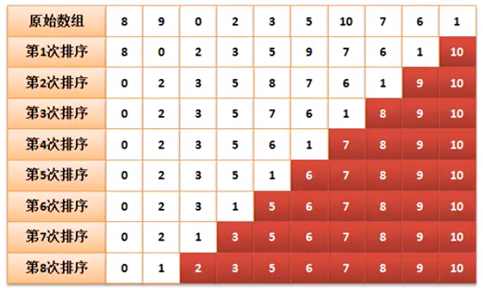public class ArrayDemo {
public static void main (String args[ ]) {
int data [] = new int [] {8,9,0,2,3,5,10,7,6,1} ;
for (int y = 0 ; y < data.length ; y ++) {
if (data[y] > data[y + 1]){
int temp = data[y] ;
data[y] = data[y + 1] ;
data[y + 1] = temp ;
}
}
printArray(data) ;
}
public static void printArray(int temp []) {
for (int x = 0 ; x < temp.lenght ; x ++) {
System.out.print (temp[x] + “、”) ;
}
System.out.println() ;
}
}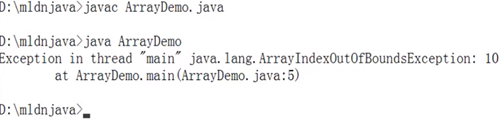for (int y = 0 ; y < data.length-1 ; y ++)public class ArrayDemo {
public static void main (String args[ ]) {
int data [] = new int [] {8,9,0,2,3,5,10,7,6,1} ;
for (int x =0 ; x < data.length ; x ++) {
for (int y = 0 ; y < data.length-1 ; y ++) {
if (data[y] > data[y + 1]){
int temp = data[y] ;
data[y] = data[y + 1] ;
data[y + 1] = temp ;
}
}
}
printArray(data) ;
}
public static void printArray(int temp []) {
for (int x = 0 ; x < temp.lenght ; x ++) {
System.out.print (temp[x] + “、”) ;
}
System.out.println() ;
}
}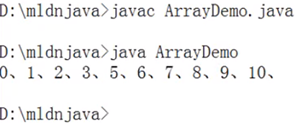for (int y = 0 ; y < data.length-x-1 ; y ++)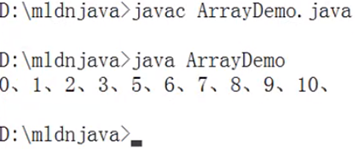class ArrayUtil {
public static void sort(int data[]) {    //进行数组的排序处理
for (int x =0 ; x < data.length ; x ++) {
for (int y = 0 ; y < data.length-x-1 ; y ++) {
if (data[y] > data[y + 1]){      //交换数据
int temp = data[y] ;
data[y] = data[y + 1] ;
data[y + 1] = temp ;
}
}
}
}
public static void printArray(int temp []) {
for (int x = 0 ; x < temp.lenght ; x ++) {
System.out.print (temp[x] + “、”) ;
}
System.out.println() ;
}
}
public class ArrayDemo {
public static void main (String args[ ]) {
int data [] = new int [] {8,9,0,2,3,5,10,7,6,1} ;
ArrayUtil.sort(data) ;         //排序
ArrayUtil.printArray(data) ;
}
}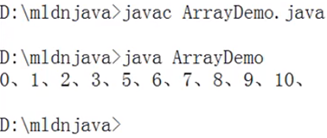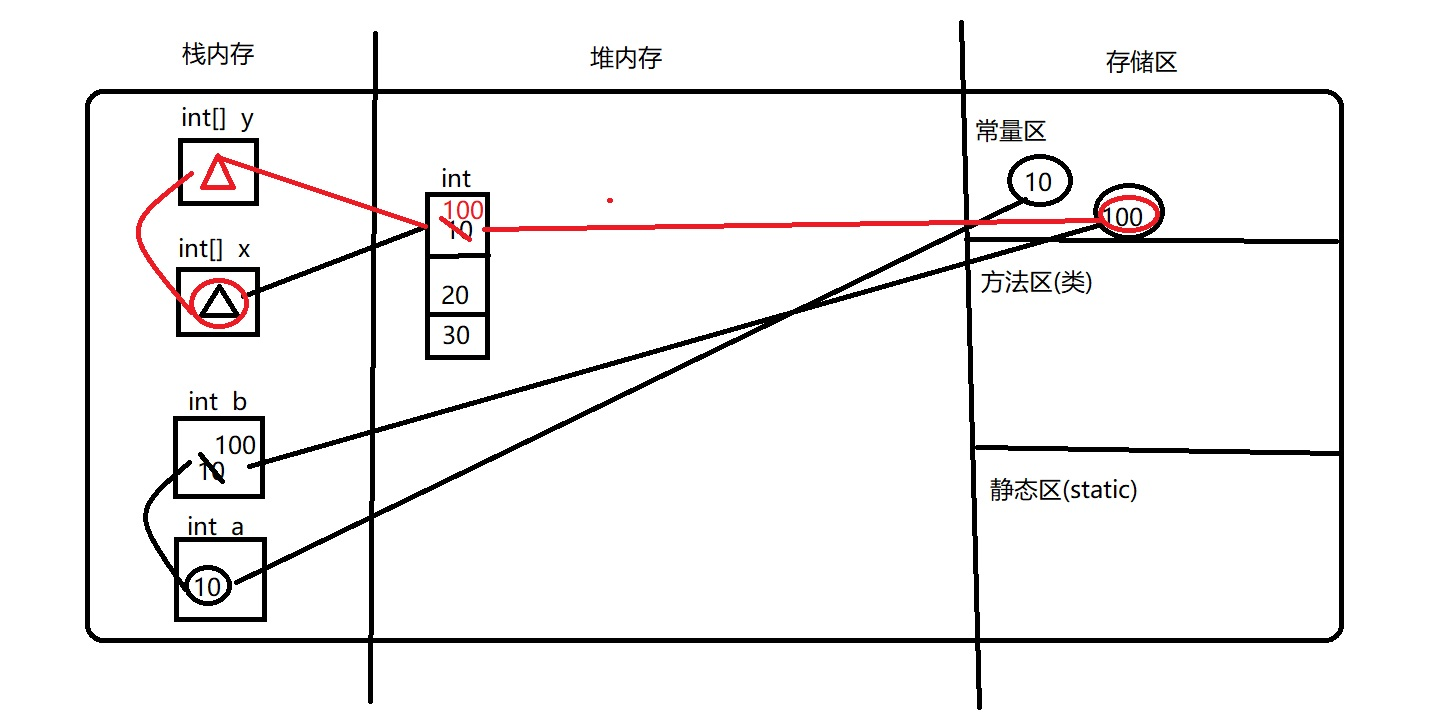Java编程之数组
# 数组(容器) 数组是一组数据类型相同的数据的组合，将这些数据统一的管理起来 数组本身是一个引用数据类型，数组内存储的类型可以是基本类型 也可以是引用类型 数组的特点 1.数组本身是一个引用数据类型 2.数组是在堆内存中的一串连续的地址存在 3.数组在初始化时必须指定长度 4.堆内存的数组空间长度一旦确定 不能再次发生改变 5.栈内存的变量中存储的是数组的地址引用 6.数组内部存储的类型可以是基本的 也可以是引用
19 0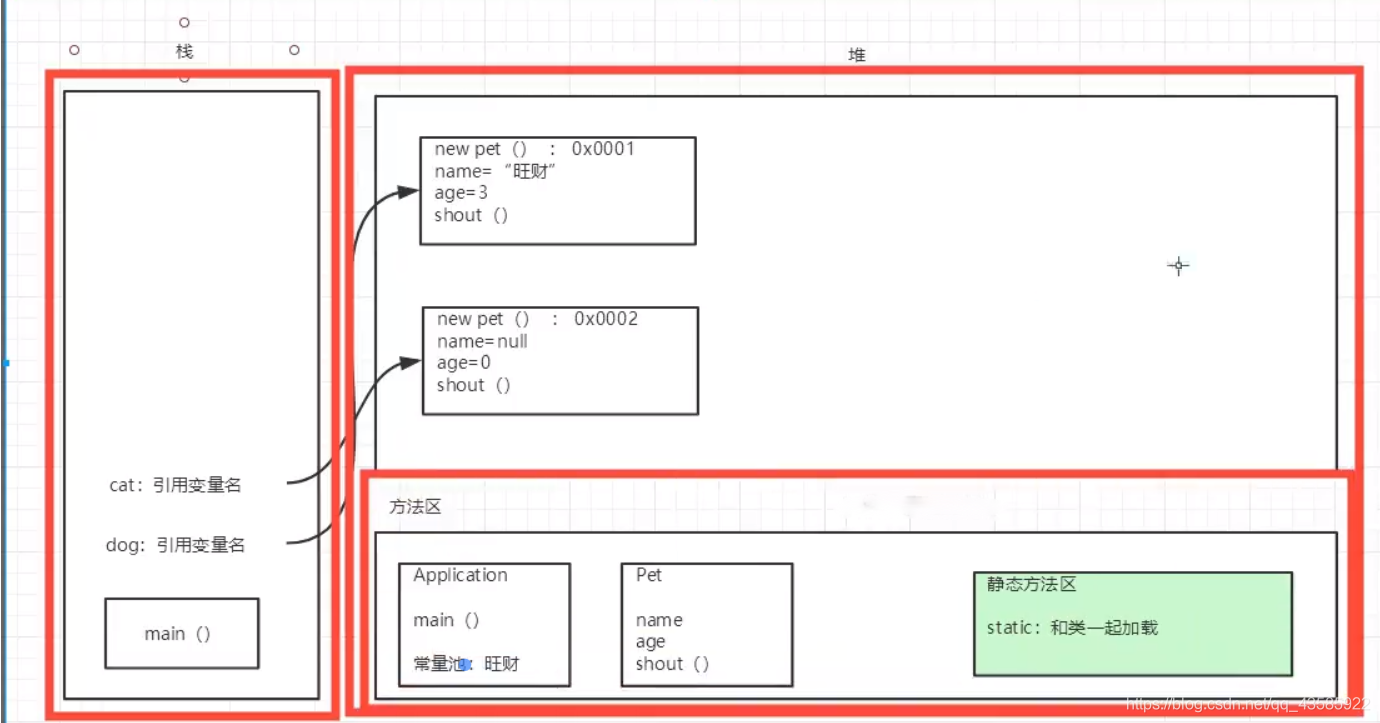Java基础——面向对象编程
Java基础——面向对象编程
36 0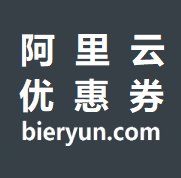639 0770 0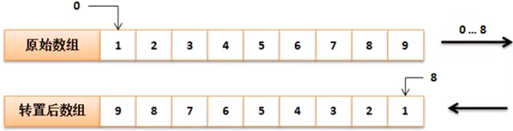2052 01500 04174 01839 0java面试-Java并发编程(九)——批量获取多条线程的执行结果

1325 0java编程之常见的排序算法
java常见的排序算法   第一种：插入排序 直接插入排序 1， 直接插入排序 （1）基本思想：在要排序的一组数中，假设前面(n-1)[n>=2] 个数已经是排 好顺序的，现在要把第n个数插到前面的有序数中，使得这n个数 也是排好顺序的。
619 0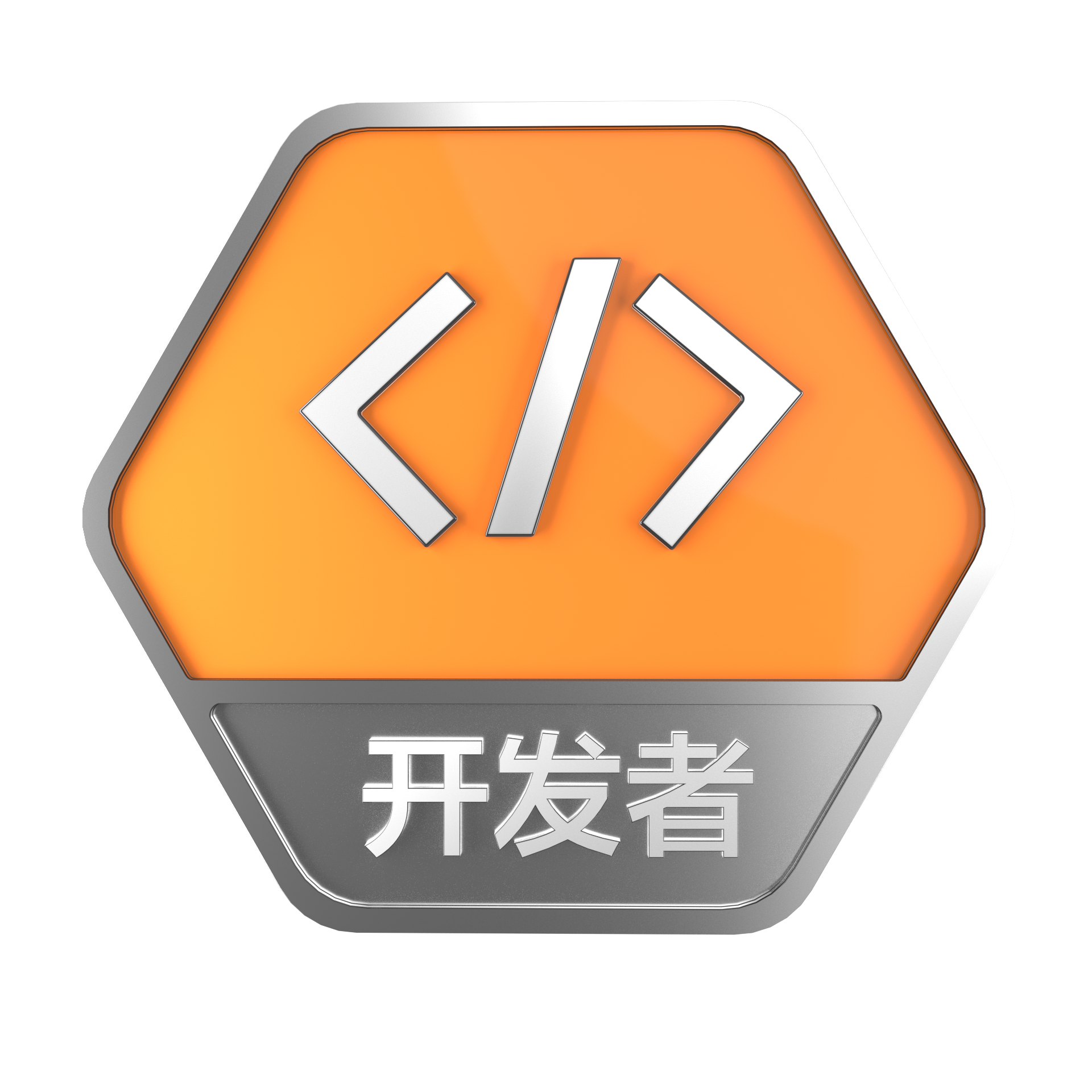+关注
1170

2

Java开发者成长课堂，课程资料学习，实战案例解析，Java工程师必备词汇等你来~
+ 订阅